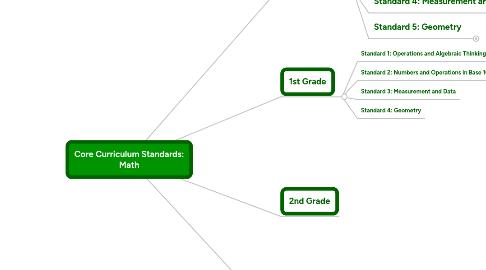# Core Curriculum Standards: Math

Get Started. It's FreeCore Curriculum Standards: Math by## 1. Kindergarten

### 1.1. Standard 1: Counting and Cardinality

1.1.1. Know number names and the count sequence

1.1.2. Count to tell the number of objects

1.1.3. Compare numbers

### 1.2. Standard 2: Operations and Algebraic Thinking

1.2.1. Understand addition as putting together and adding to, and understand subtraction as taking apart and taking from.

### 1.3. Standard 3: Number & Operations in Base Ten

1.3.1. Work with numbers 11-19 to gain foundations for place value

### 1.4. Standard 4: Measurement and Data

1.4.1. Describe and compare measurable attributes.

1.4.2. Classify objects and count the number of objects in each category.

### 1.5. Standard 5: Geometry

1.5.1. Identify and describe shapes (squares, circles, triangles, rectangles, hexagons, cubes, cones, cylinders, and spheres).

1.5.2. Analyze, compare, create, and compose shapes.